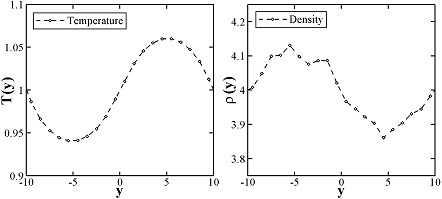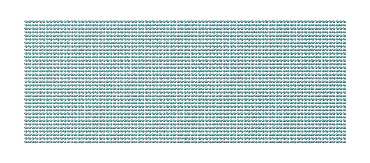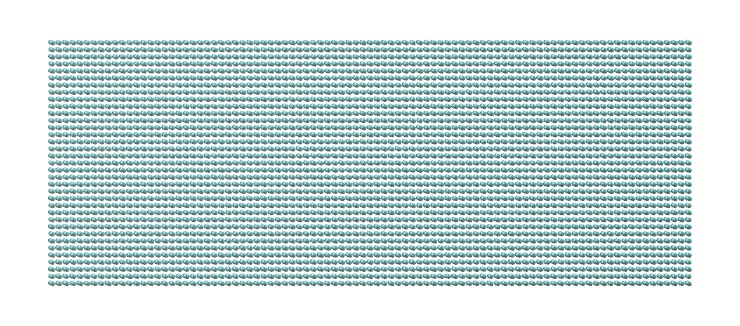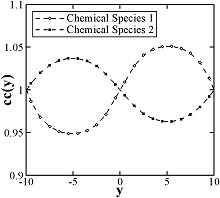$$\renewcommand{\AA}{\text{Å}}$$

# pair_style tdpd command

## Syntax

pair_style style args

• style = edpd or mdpd or mdpd/rhosum or tdpd

• args = list of arguments for a particular style

edpd args = cutoff seed
cutoff = global cutoff for eDPD interactions (distance units)
seed = random # seed (integer) (if <= 0, eDPD will use current time as the seed)
mdpd args = T cutoff seed
T = temperature (temperature units)
cutoff = global cutoff for mDPD interactions (distance units)
seed = random # seed (integer) (if <= 0, mDPD will use current time as the seed)
mdpd/rhosum args =
tdpd args = T cutoff seed
T = temperature (temperature units)
cutoff = global cutoff for tDPD interactions (distance units)
seed = random # seed (integer) (if <= 0, tDPD will use current time as the seed)

## Examples

pair_style edpd 1.58 9872598
pair_coeff * * 18.75 4.5 0.41 1.58 1.42E-5 2.0 1.58
pair_coeff 1 1 18.75 4.5 0.41 1.58 1.42E-5 2.0 1.58 power 10.54 -3.66 3.44 -4.10
pair_coeff 1 1 18.75 4.5 0.41 1.58 1.42E-5 2.0 1.58 power 10.54 -3.66 3.44 -4.10 kappa -0.44 -3.21 5.04 0.00

pair_style hybrid/overlay mdpd/rhosum mdpd 1.0 1.0 65689
pair_coeff 1 1 mdpd/rhosum  0.75
pair_coeff 1 1 mdpd -40.0 25.0 18.0 1.0 0.75

pair_style tdpd 1.0 1.58 935662
pair_coeff * * 18.75 4.5 0.41 1.58 1.58 1.0 1.0E-5 2.0
pair_coeff 1 1 18.75 4.5 0.41 1.58 1.58 1.0 1.0E-5 2.0 3.0 1.0E-5 2.0


## Description

The edpd style computes the pairwise interactions and heat fluxes for eDPD particles following the formulations in (Li2014_JCP) and Li2015_CC. The time evolution of an eDPD particle is governed by the conservation of momentum and energy given by

$\begin{split}\frac{\mathrm{d}^2 \mathbf{r}_i}{\mathrm{d} t^2}= \frac{\mathrm{d} \mathbf{v}_i}{\mathrm{d} t} =\mathbf{F}_{i}=\sum_{i\neq j}(\mathbf{F}_{ij}^{C}+\mathbf{F}_{ij}^{D}+\mathbf{F}_{ij}^{R}) \\ C_v\frac{\mathrm{d} T_i}{\mathrm{d} t}= q_{i} = \sum_{i\neq j}(q_{ij}^{C}+q_{ij}^{V}+q_{ij}^{R}),\end{split}$

where the three components of $$F_{i}$$ including the conservative force $$F_{ij}^C$$, dissipative force $$F_{ij}^D$$ and random force $$F_{ij}^R$$ are expressed as

$\begin{split}\mathbf{F}_{ij}^{C} & = \alpha_{ij}{\omega_{C}}(r_{ij})\mathbf{e}_{ij} \\ \mathbf{F}_{ij}^{D} & = -\gamma {\omega_{D}}(r_{ij})(\mathbf{e}_{ij} \cdot \mathbf{v}_{ij})\mathbf{e}_{ij} \\ \mathbf{F}_{ij}^{R} & = \sigma {\omega_{R}}(r_{ij}){\xi_{ij}}\Delta t^{-1/2} \mathbf{e}_{ij} \\ \omega_{C}(r) & = 1 - r/r_c \\ \alpha_{ij} & = A\cdot k_B(T_i + T_j)/2 \\ \omega_{D}(r) & = \omega^2_{R}(r) = (1-r/r_c)^s \\ \sigma_{ij}^2 & = 4\gamma k_B T_i T_j/(T_i + T_j)\end{split}$

in which the exponent of the weighting function s can be defined as a temperature-dependent variable. The heat flux between particles accounting for the collisional heat flux $$q^C$$, viscous heat flux $$q^V$$, and random heat flux $$q^R$$ are given by

$\begin{split}q_i^C & = \sum_{j \ne i} k_{ij} \omega_{CT}(r_{ij}) \left( \frac{1}{T_i} - \frac{1}{T_j} \right) \\ q_i^V & = \frac{1}{2 C_v}\sum_{j \ne i}{ \left\{ \omega_D(r_{ij})\left[\gamma_{ij} \left( \mathbf{e}_{ij} \cdot \mathbf{v}_{ij} \right)^2 - \frac{\left( \sigma _{ij} \right)^2}{m}\right] - \sigma _{ij} \omega_R(r_{ij})\left( \mathbf{e}_{ij} \cdot \mathbf{v}_{ij} \right){\xi_{ij}} \right\} } \\ q_i^R & = \sum_{j \ne i} \beta _{ij} \omega_{RT}(r_{ij}) d {t^{ - 1/2}} \xi_{ij}^e \\ \omega_{CT}(r) & =\omega_{RT}^2(r)=\left(1-r/r_{ct}\right)^{s_T} \\ k_{ij} & =C_v^2\kappa(T_i + T_j)^2/4k_B \\ \beta_{ij}^2 & = 2k_Bk_{ij}\end{split}$

where the mesoscopic heat friction $$\kappa$$ is given by

$\kappa = \frac{315k_B\upsilon }{2\pi \rho C_v r_{ct}^5}\frac{1}{Pr},$

with $$\upsilon$$ being the kinematic viscosity. For more details, see Eq.(15) in (Li2014_JCP).

The following coefficients must be defined in eDPD system for each pair of atom types via the pair_coeff command as in the examples above.

• A (force units)

• $$\gamma$$ (force/velocity units)

• power_f (positive real)

• cutoff (distance units)

• kappa (thermal conductivity units)

• power_T (positive real)

• cutoff_T (distance units)

• optional keyword = power or kappa

The keyword power or kappa is optional. Both “power” and “kappa” require 4 parameters $$c_1, c_2, c_3, c_4$$ showing the temperature dependence of the exponent $$s(T) = \mathrm{power}_f ( 1+c_1 (T-1) + c_2 (T-1)^2 + c_3 (T-1)^3 + c_4 (T-1)^4 )$$ and of the mesoscopic heat friction $$s_T(T) = \kappa (1 + c_1 (T-1) + c_2 (T-1)^2 + c_3 (T-1)^3 + c_4 (T-1)^4)$$. If the keyword power or kappa is not specified, the eDPD system will use constant power_f and $$\kappa$$, which is independent to temperature changes.

The mdpd/rhosum style computes the local particle mass density $$\rho$$ for mDPD particles by kernel function interpolation.

The following coefficients must be defined for each pair of atom types via the pair_coeff command as in the examples above.

• cutoff (distance units)

The mdpd style computes the many-body interactions between mDPD particles following the formulations in (Li2013_POF). The dissipative and random forces are in the form same as the classical DPD, but the conservative force is local density dependent, which are given by

$\begin{split}\mathbf{F}_{ij}^C & = Aw_c(r_{ij})\mathbf{e}_{ij} + B(\rho_i+\rho_j)w_d(r_{ij})\mathbf{e}_{ij} \\ \mathbf{F}_{ij}^{D} & = -\gamma {\omega_{D}}(r_{ij})(\mathbf{e}_{ij} \cdot \mathbf{v}_{ij})\mathbf{e}_{ij} \\ \mathbf{F}_{ij}^{R} & = \sigma {\omega_{R}}(r_{ij}){\xi_{ij}}\Delta t^{-1/2} \mathbf{e}_{ij}\end{split}$

where the first term in $$F_C$$ with a negative coefficient $$A < 0$$ stands for an attractive force within an interaction range $$r_c$$, and the second term with $$B > 0$$ is the density-dependent repulsive force within an interaction range $$r_d$$.

The following coefficients must be defined for each pair of atom types via the pair_coeff command as in the examples above.

• A (force units)

• B (force units)

• $$\gamma$$ (force/velocity units)

• cutoff_c (distance units)

• cutoff_d (distance units)

The tdpd style computes the pairwise interactions and chemical concentration fluxes for tDPD particles following the formulations in (Li2015_JCP). The time evolution of a tDPD particle is governed by the conservation of momentum and concentration given by

$\begin{split}\frac{\mathrm{d}^2 \mathbf{r}_i}{\mathrm{d} t^2} & = \frac{\mathrm{d} \mathbf{v}_i}{\mathrm{d} t}=\mathbf{F}_{i}=\sum_{i\neq j}(\mathbf{F}_{ij}^{C}+\mathbf{F}_{ij}^{D}+\mathbf{F}_{ij}^{R}) \\ \frac{\mathrm{d} C_{i}}{\mathrm{d} t} & = Q_{i} = \sum_{i\neq j}(Q_{ij}^{D}+Q_{ij}^{R}) + Q_{i}^{S}\end{split}$

where the three components of $$F_{i}$$ including the conservative force $$F_{ij}^C$$, dissipative force $$F_{ij}^C$$ and random force $$F_{ij}^C$$ are expressed as

$\begin{split}\mathbf{F}_{ij}^{C} & = A{\omega_{C}}(r_{ij})\mathbf{e}_{ij} \\ \mathbf{F}_{ij}^{D} & = -\gamma {\omega_{D}}(r_{ij})(\mathbf{e}_{ij} \cdot \mathbf{v}_{ij})\mathbf{e}_{ij} \\ \mathbf{F}_{ij}^{R} & = \sigma {\omega_{R}}(r_{ij}){\xi_{ij}}\Delta t^{-1/2} \mathbf{e}_{ij} \\ \omega_{C}(r) & = 1 - r/r_c \\ \omega_{D}(r) & = \omega^2_{R}(r) = (1-r/r_c)^{\rm power_f} \\ \sigma^2 = 2\gamma k_B T\end{split}$

The concentration flux between two tDPD particles includes the Fickian flux $$Q_{ij}^D$$ and random flux $$Q_{ij}^R$$, which are given by

$\begin{split}Q_{ij}^D & = -\kappa_{ij} w_{DC}(r_{ij}) \left( C_i - C_j \right) \\ Q_{ij}^R & = \epsilon_{ij}\left( C_i + C_j \right) w_{RC}(r_{ij}) \xi_{ij} \\ w_{DC}(r_{ij}) & =w^2_{RC}(r_{ij}) = (1 - r/r_{cc})^{\rm power_{cc}} \\ \epsilon_{ij}^2 & = m_s^2\kappa_{ij}\rho\end{split}$

where the parameters kappa and epsilon determine the strength of the Fickian and random fluxes. $$m_s$$ is the mass of a single solute molecule. In general, $$m_s$$ is much smaller than the mass of a tDPD particle m. For more details, see (Li2015_JCP).

The following coefficients must be defined for each pair of atom types via the pair_coeff command as in the examples above.

• A (force units)

• $$\gamma$$ (force/velocity units)

• power_f (positive real)

• cutoff (distance units)

• cutoff_CC (distance units)

• $$\kappa_i$$ (diffusivity units)

• $$\epsilon_i$$ (diffusivity units)

• power_cc_i (positive real)

The last 3 values must be repeated Nspecies times, so that values for each of the Nspecies chemical species are specified, as indicated by the “I” suffix. In the first pair_coeff example above for pair_style tdpd, Nspecies = 1. In the second example, Nspecies = 2, so 3 additional coeffs are specified (for species 2).

## Example scripts

There are example scripts for using all these pair styles in examples/PACKAGES/mesodpd. The example for an eDPD simulation models heat conduction with source terms analog of periodic Poiseuille flow problem. The setup follows Fig.12 in (Li2014_JCP). The output of the short eDPD simulation (about 2 minutes on a single core) gives a temperature and density profiles asThe example for a mDPD simulation models the oscillations of a liquid droplet started from a liquid film. The mDPD parameters are adopted from (Li2013_POF). The short mDPD run (about 2 minutes on a single core) generates a particle trajectory which can be visualized as follows.The first image is the initial state of the simulation. If you click it a GIF movie should play in your browser. The second image is the final state of the simulation.

The example for a tDPD simulation computes the effective diffusion coefficient of a tDPD system using a method analogous to the periodic Poiseuille flow. The tDPD system is specified with two chemical species, and the setup follows Fig.1 in (Li2015_JCP). The output of the short tDPD simulation (about one and a half minutes on a single core) gives the concentration profiles of the two chemical species as## Mixing, shift, table, tail correction, restart, rRESPA info

The styles edpd, mdpd, mdpd/rhosum and tdpd do not support mixing. Thus, coefficients for all I,J pairs must be specified explicitly.

The styles edpd, mdpd, mdpd/rhosum and tdpd do not support the pair_modify shift, table, and tail options.

The styles edpd, mdpd, mdpd/rhosum and tdpd do not write information to binary restart files. Thus, you need to re-specify the pair_style and pair_coeff commands in an input script that reads a restart file.

## Restrictions

The pair styles edpd, mdpd, mdpd/rhosum and tdpd are part of the DPD-MESO package. They are only enabled if LAMMPS was built with that package. See the Build package page for more info.

## Default

none

(Li2014_JCP) Li, Tang, Lei, Caswell, Karniadakis, J Comput Phys, 265: 113-127 (2014). DOI: 10.1016/j.jcp.2014.02.003.

(Li2015_CC) Li, Tang, Li, Karniadakis, Chem Commun, 51: 11038-11040 (2015). DOI: 10.1039/C5CC01684C.

(Li2013_POF) Li, Hu, Wang, Ma, Zhou, Phys Fluids, 25: 072103 (2013). DOI: 10.1063/1.4812366.

(Li2015_JCP) Li, Yazdani, Tartakovsky, Karniadakis, J Chem Phys, 143: 014101 (2015). DOI: 10.1063/1.4923254.